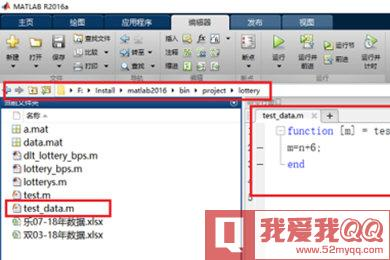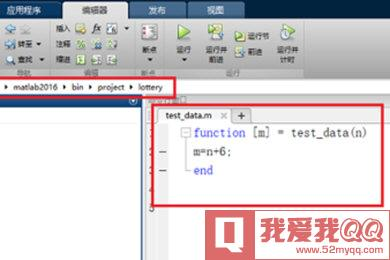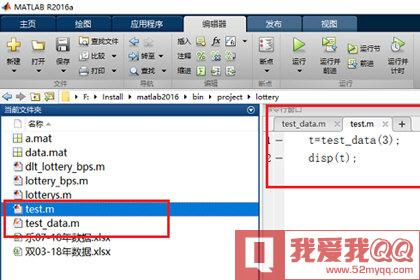• Matlab如何调用其他m文件中的函数
千次阅读
2021-04-18 07:07:06

我们若可以根据需要建立自己的函数文件,他们能够像库函数一样方便地调用,从而极大地扩展MATLAB地能力.

如果对于一类特殊地问题,建立起来许多函数M文件,就能最终形成独立地工具箱.

1.函数M文件地基本用法

function 因变量=函数名 (自变量)

其它各行为从自变量计算因变量地语句,并最终将结果赋予因变量.

这个M文件地文件名最好是函数名.m

function a = randint (m, n)

% RANDINT Randomly generated integral matrix

a = floor (10*rand(m,n))

当需要一个这样地2*3随机矩阵时,只需

>>x=randint(2,3)

x= 9 6 8

2 4 7

2.函数M文件有多个因变量

要用[]将他们括起来,请看下例:

function [mean, stdev] = stat(x)

% STAT Mean and standard deviation

[m n] = size(x);

if m =1

m=n

end

mean= sum(x)/m;

stdev=sqrt(sum(x^2)/m-mean^2)

其用途,如求一个数组X地平均值和均方差,只需:

>>x= [ 2 4 -7 0 5 -1]；

[xm.xd]=stat(x)

xm=0. 5

xd=3.9476

更多相关内容
• MATLAB调用refprop，里面包含四个除dll外所需要的文件
• 函数将创建一个树结构，其中包含作为输入给出的 m 文件中的所有函数调用。 该函数还将自动搜索被调用的 m 文件调用自身的函数不会创建无限循环。 您可以设置'搜索区域'，fi 该功能将只搜索'.\work\' 中的m 文件...
• PAGE / NUMPAGES Matlab 自定义函数 ?1函数文件+调用命令文件需单独定义一个自定义函数的M文件; ?2函数文件+子函数定义一个具有多个自定义函数的M文件 ?3Inline:无需M文件直接定义 ?...调用函数文件:my
• 1、函数文件的基本结构 function 输出形参表=函数名（输入形参表) 注释说明部分 函数体语句 当有多个形参时，形参之间用...在调用函数时，函数输入输出参数称为实际参数，简称实参。 例1：编写函数文件,求半径为r的圆的

1、函数文件的基本结构

function 输出形参表=函数名（输入形参表)
注释说明部分
函数体语句

当有多个形参时，形参之间用逗号分隔，组成形参表。当输出形参多于一个时，应该用方括号括起来，构成一个输出矩阵。

函数文件名和函数名：
（1）函数文件名通常由函数名再加上扩展名.m组成,函数文件名与函数名也可以不相同。
（2）return语句表示结束函数的执行。

函数调用
调用格式:
[输出实参表=函数名(输入实参表)

在调用函数时，函数输入输出参数称为实际参数，简称实参。

例1：编写函数文件,求半径为r的圆的面积和周长。

function [S,C] = circle(r)
S = pi*r*r;  %圆的面积
C = 2*pi*r;  %圆的周长

%在命令行窗口调用函数
>> [S,C] = circle(10)

%输出结果
S =
314.1593

C =
62.831


2、匿名函数
基本格式:

（1）函数句柄变量=@(匿名函数输入参数)匿名函数表达式
其中@为函数句柄的运算符

>> f = @(x,y)x^2+y^2

f =

包含以下值的 function_handle:

@(x,y)x^2+y^2

%输出结果
>> f(1,2)
>
ans =
5


（2）函数句柄变量=@函数名
函数名可为：内部函数或自定义函数

>> f = @sind

f =

包含以下值的 function_handle:

@sind

>> f(30)

ans =
0.5000%用匿名函数定义f
f = @(n)n+10*log(n*n+5);
y = f(40)/[f(30)+f(20)]

%输出结果
y =
0.6390

%用函数文件定义函数f
function y = f2(n)
y = 0;
for i=1:n
y = y + i*(i+1);
end

%在命令行窗口调用f2函数，其输出结果
>> f2(40)/[f2(30)+f2(20)]

ans =
1.7662

展开全文• 那么来看如何在脚本文件里调用函数文件中的函数。 注意点： 　注意相应的格式问题，函数文件就是以函数名称命名的，暂时不知道可否在一个函数文件里放多个函数（引发如何命名的问题）。 例子代码： 　 1...

问题：

matlab里面有两种文件，一种是脚本文件，一种是函数文件，为了模块化程序，我们需要把专门的功能写成一个函数封装到某个函数文件里面。

那么来看如何在脚本文件里调用函数文件中的函数。

注意点：

注意相应的格式问题，函数文件就是以函数名称命名的，暂时不知道可否在一个函数文件里放多个函数（引发如何命名的问题）。

例子代码：1 % matlab脚本文件
2
3 myFun(1,1)        % 注意，加分号则不会马上执行得到值，不加分号可以打印表达式的值
main.m1 % matlab函数文件
2 % 求和函数,测试matlab函数功能
3
4 function y = myFun( a, b )
5     y = a+b;
6 end
myFun.m

就是这么简单。

转载于:https://www.cnblogs.com/jerrybaby/p/6141567.html

展开全文• 想要用WPF调用Matlab代码，就用到了Matlab生成.DLL文件的功能。 注：我的VS版本是2013，Matlab版本是2012a，两个软件不算新也不算老，应该是现在普遍用的版本 首先，生成Matlab的.DLL文件matlab的左下角选择start...
• 今天小编就为大家分享一篇python调用matlab的m自定义函数方法，具有很好的参考价值，希望对大家有所帮助。一起跟随小编过来看看吧
• 采用matlab软件中的m文件实现，分段函数示例， 文档中有相应的注释，方便大家学习。
• Matlab作为一款专业性极强的商业数学软件，将诸多的...调用函数：首先我们需要在Matlab中打开New M-File新建M文件；再创建一个自定义函数test_data，并将test_data函数文件放在当前文件夹内；在命令行窗口中输入简单...

Matlab作为一款专业性极强的商业数学软件，将诸多的算法开发、统计分析、数据可视化功能融入其中，用户可以方便地调用需要的函数，建立数学模型，为了满足你工作的需要，还可以自行设置自己需要的函数，下面就跟小编了解下吧。调用函数：

首先我们需要在Matlab中打开New M-File新建M文件；

再创建一个自定义函数test_data，并将test_data函数文件放在当前文件夹内；在命令行窗口中输入简单定义函数test_data(3)，返回结果；

然后在命令行窗口中调用函数，需要注意的是调用函数要在当前文件夹中，就是要在自定义函数test_data的路径文件夹下，不然会出现找不到的情况，根本无法调用。前面小编已经将函数放在当前文件夹，现在只需要前往确认下即可；

另外我们也可以创建M脚本文件来调用自定义函数，在M脚本文件输入：

t=test_data(3);

disp(t);需要注意的是脚本文件和自定义函数文件必须在同一个文件夹下；

最后我们运行脚本文件就可以在命令行得出结果，看看是不是你想要调用的函数吧。

以上就是小编为你准备的Matlab自定义函数调用方法，如果你编写的函数文件是日常工作中经常会用到的，可以将函数文件放入指定位置后(这里必须重新预设路径)，并保存，就可以在需要的时候快速调用了。

展开全文• Matlab 调用refprop（64位环境）和物性函数..
• Matlab的.m文件生成动态链接库，然后在VS的工程项目中调用动态链接库。可以完美实现VC调用Matlab函数
• 小编今天带来的是关于Matlab调用函数的详细操作，下面就一起来学习关于Matlab调用函数的操作步骤吧，希望能够帮助到大家。Matlab调用函数的详细操作首先我们需要在Matlab中打开New M-File新建M文件;再创建一个自定义...
• ## MATLAB函数调用

千次阅读 2021-04-15 19:17:44
1、首先建立M函数文件右键——新建——函数，其中函数文件的格式、是： function [输出变量] = 函数名称(输入变量） 如下所示，是编写的一个求1到n之和的求和函数 A，按照上述格式，编写代码如下并保存文件，注意...
• Matlab作为一款专业性极强的商业数学软件，将诸多的...调用函数：首先我们需要在Matlab中打开New M-File新建M文件；再创建一个自定义函数test_data，并将test_data函数文件放在当前文件夹内；在命令行窗口中输入简单...
• QT调用第三方MATLAB库和函数使用-附件资源
• Matlab作为一款专业性极强的商业数学软件，将诸多的...调用函数：首先我们需要在Matlab中打开New M-File新建M文件；再创建一个自定义函数test_data，并将test_data函数文件放在当前文件夹内；在命令行窗口中输入简单...
• 简单的饱和函数m文件，在matlab下可以调用，实现matlab下运行饱和函数的作用
• ## matlab中创建和调用m文件

万次阅读 多人点赞 2018-07-13 20:11:19
2、函数文件，第一行为function ，比如说是function y = name(x)这种文件，函数名与文件名是一致的，在命令窗口里输入name(x),x是运行参数，回车即运行。其中以function开头的一行为引导行，表...
• matlab 定义函数,matlab定义函数调用,Matlab自定义函数详解很久以前写的一篇Matlab自定义函数访问量很大，可惜没有点赞的，我感觉是我没讲清楚，这里又写了一篇笔记Matlab函数函数是组织好的，可重复使用的，用来...
• 函数 find_references 搜索当前文件夹中的 matlab 文件以查找调用/引用某个函数文件。 文件名，包括指向显示函数的行的超链接（见截图） find_references（函数名） 例子find_references('disp'); 注意，这个...
• ## MATLAB调用M文件

千次阅读 2020-05-17 20:24:40
MATLAB调用M文件 有一个朋友问我怎么用MATLAB调用M文件，我也只是个新手，这点还碰巧会，就告诉了他。想着分享给大家， 采用调用函数的方式 首先创建一个.以函数名为名字的m文件，然后定义自己想用的函数，我这里...
• 目录一、建立文件二、函数调用二、函数句柄三、匿名函数想查找相关内容也可在页面按ctrl+F搜索文字一、建立文件新建脚本，保存后可运行，后缀为.m 二、函数调用创建ffib.m文件，编写求Fibonacci数列的函数function...
• matlab function m文件 全局变量调用 可以用于模拟c 验证函数功能 以及验证算法正确性
• ## Matlab函数文件简介

千次阅读 2021-04-25 13:30:22
matlab中，M文件分为脚本文件和函数文件。如果M文件的第一个可执行语句以function开头，那么这个M文件就是函数文件。 脚本文件与函数文件的区别 脚本文件只是存储在文件中的 matlab 语句的集合。执行脚本文件时，...
• .m文件，就是ftx功能函数调用，后面不知道怎么形容。...

# matlab怎么调用函数文件matlab 订阅